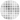# Control color in seaborn heatmaps

While you can plot a basic heatmap and make basic customizations using seaborn library, you can also control the color palette of your graph. This is a crucial step since the choice of colors may affect the message given by your heatmap.

Changing the color palette of a seaborn heatmap is expalined with examples in 3 sections below. Note that datacamp offers this online course to understand the basics of seaborn.

## Sequential Palette : one color only

You can customize the colors in your heatmap with the `cmap` parameter of the `heatmap()` function in seaborn. The following examples show the appearences of different sequential color palettes.

``````# libraries
import seaborn as sns
import matplotlib.pyplot as plt
import pandas as pd
import numpy as np

# Create a dataset
df = pd.DataFrame(np.random.random((10,10)), columns=["a","b","c","d","e","f","g","h","i","j"])

# plot using a color palette
sns.heatmap(df, cmap="YlGnBu")
plt.show()

sns.heatmap(df, cmap="Blues")
plt.show()

sns.heatmap(df, cmap="BuPu")
plt.show()

sns.heatmap(df, cmap="Greens")
plt.show()``````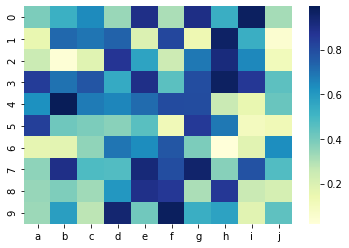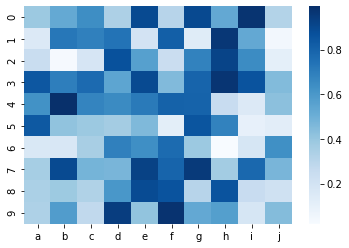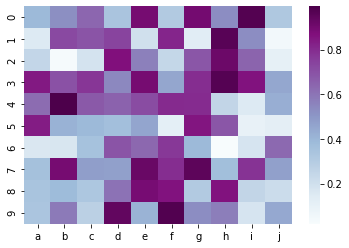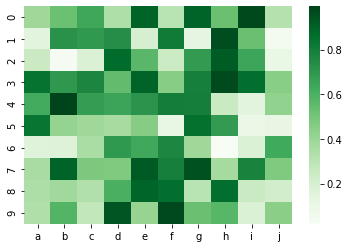It is also possible to set maximum and minimum values for color bar on a seaborn heatmap by giving values to `vmax` and `vmin` parameters in the function.

``````# libraries
import seaborn as sns
import matplotlib.pyplot as plt
import pandas as pd
import numpy as np

# Create a dataset
df = pd.DataFrame(np.random.random((10,10)), columns=["a","b","c","d","e","f","g","h","i","j"])

# color bar range between 0 and 0.5
sns.heatmap(df, vmin=0, vmax=0.5)
plt.show()

# color bar range between 0.5 and 0.7
sns.heatmap(df, vmin=0.5, vmax=0.7)
plt.show()``````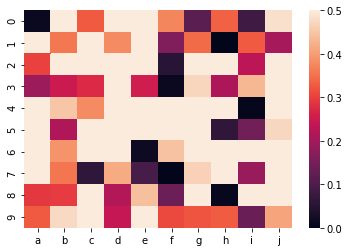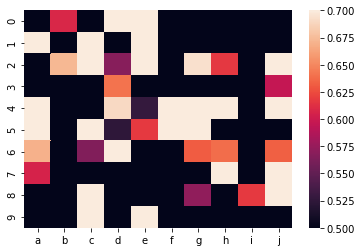## Diverging Palette : two contrasting colors

Following example uses 2 contrast colors pink and yellow-green in the heatmap.

``````# libraries
import seaborn as sns
import matplotlib.pyplot as plt
import pandas as pd
import numpy as np

# create dataset
df = np.random.randn(30, 30)

# plot heatmap
sns.heatmap(df, cmap="PiYG")
plt.show()``````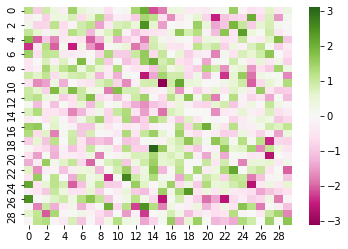When plotting divergant data, you can specify the value at which to center the colormap using `center` parameter. You can see the following example heatmap for data centered on 1 with a diverging colormap:

``````# libraries
import seaborn as sns
import matplotlib.pyplot as plt
import pandas as pd
import numpy as np

# create dataset
df = np.random.randn(30, 30)

# plot heatmap
sns.heatmap(df, center=1)
plt.show()``````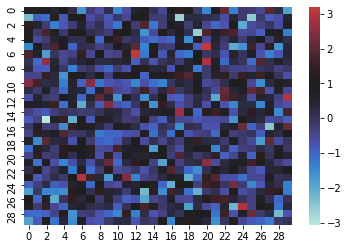## Discrete Data

If your dataset consists of continues values, you can turn them into discrete numbers and use these discrete values in the heatmap. The following examples shows how to transform continues values into 3 discrete values: 0, 1, and 2.

``````# libraries
import seaborn as sns
import matplotlib.pyplot as plt
import pandas as pd
import numpy as np

# create data
df = pd.DataFrame(np.random.randn(6, 6))

# make the values in dataset discrete
# the values will be cut into 3 discrete values: 0,1,2
df_q = pd.DataFrame()
for col in df:
df_q[col] = pd.to_numeric( pd.qcut(df[col], 3, labels=list(range(3))) )

# plot it
sns.heatmap(df_q)
plt.show()``````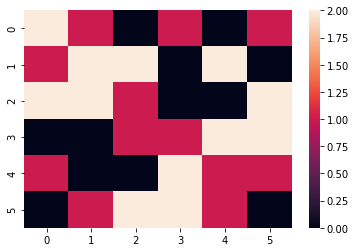## Contact & Edit

👋 This document is a work by Yan Holtz. Any feedback is highly encouraged. You can fill an issue on Github, drop me a message onTwitter, or send an email pasting `yan.holtz.data` with `gmail.com`.